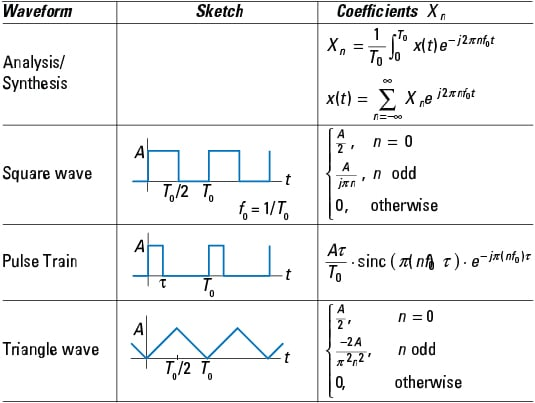##### Signals and Systems For Dummies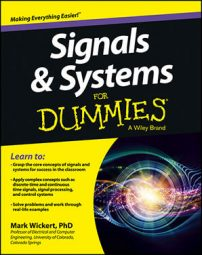Signals and systems is an aspect of electrical engineering that applies mathematical concepts to the creation of product design, such as cell phones and automobile cruise control systems. Absorbing the core concepts of signals and systems requires a firm grasp on their properties and classifications; a solid knowledge of algebra, trigonometry, complex arithmetic, calculus of one variable; and familiarity with linear constant coefficient (LCC) differential equations.

## Getting to know the mathematical foundation of signals and systems

The study of signals and systems establishes a mathematical formalism for analyzing, modeling, and simulating electrical systems in the time, frequency, and s or zdomains. Signals exist naturally and are also created by people. Some operate continuously (known as continuous-time signals); others are active at specific instants of time (and are called discrete-time signals).

Signals pass through systems to be modified or enhanced in some way. Systems that operate on signals are also categorized as continuous- or discrete-time.

Mathematics plays a central role in all facets of signals and systems. Specifically, complex arithmetic, trigonometry, and geometry are mainstays of this dynamic and (ahem) electrifying field of work and study. This article highlights the most applicable concepts from each of these areas of math for signals and systems work.

## Complex arithmetic for signals and systems

Here are some of the most important complex arithmetic operations and formulas that relate to signals and systems.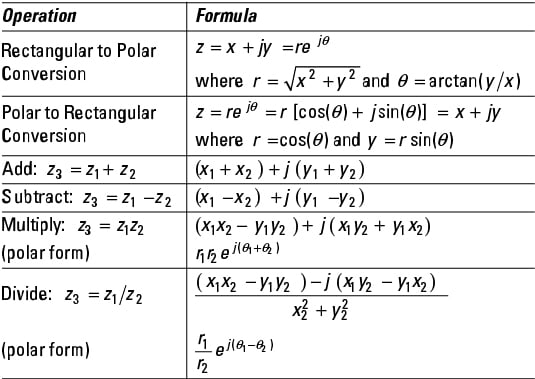## Trigonometry and Euler’s formulas

This table presents the key formulas of trigonometry that apply to signals and systems: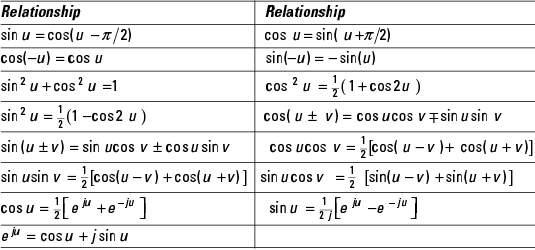## Geometric series

Among the most important geometry equations to know for signals and systems are these three: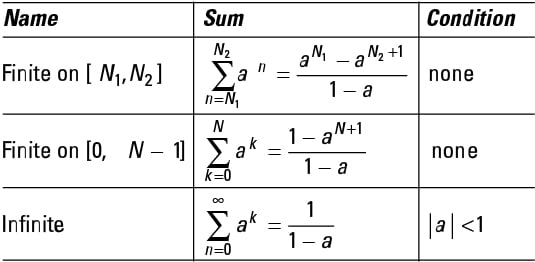## Recognizing signal properties and classifications

Signals — both continuous-time signals and their discrete-time counterparts — are categorized according to certain properties, such as deterministic or random, periodic or aperiodic, power or energy, and even or odd. These traits aren’t mutually exclusive; signals can hold multiple classifications.

Here are some of the most important signal properties.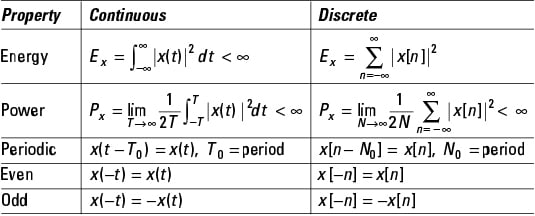But wait! There’s more. Signals can also be categorized as exponential, sinusoidal, or a special sequence. The unit sample sequence and the unit step sequence are special signals of interest in discrete-time. All the continuous-time signal classifications have discrete-time counterparts, except singularity functions, which appear in continuous-time only.

Defining special signals that serve as building blocks for more complex signals makes the creation of custom signal models to suit your needs more systematic and convenient.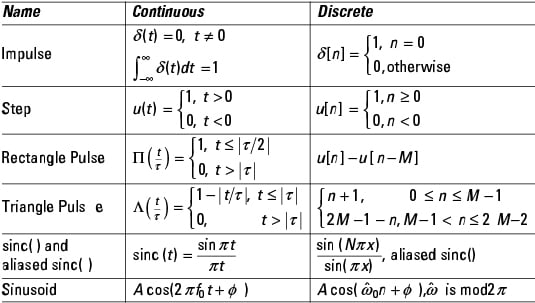## Recognizing system properties and classifications

Part of learning about signals and systems is that systems are identified according to certain properties they exhibit. Have a look at the core system classifications:

• Linearity: A linear combination of individually obtained outputs is equivalent to the output obtained by the system operating on the corresponding linear combination of inputs.

• Time-invariant: The system properties don’t change with time. A present input produces the same response as it does in the future, less the time shift factor between the present and future.

• Memoryless: If the present system output depends only on the present input, the system is memoryless.

• Causal: The present system output depends at most on the present and past inputs. Future inputs can’t be used to produce the present output.

• Stable: A system is bounded-input bound-output (BIBO) stable if all bounded inputs produce a bounded output.

This table presents core linear time invariant (LTI) system properties for both continuous and discrete-time systems. Time-domain, frequency-domain, and s/z-domain properties are identified for the categories basic input/output, cascading, linear constant coefficient (LCC) differential and difference equations, and BIBO stability: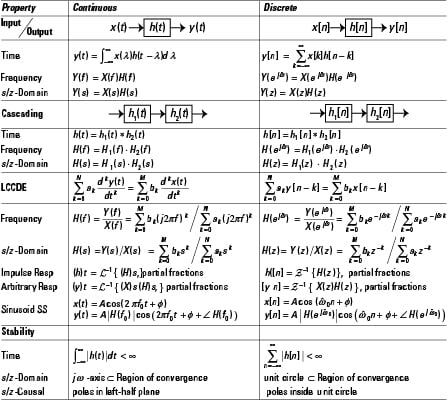## Signals and systems: working with transform theorems and pairs

Both signals and systems can be analyzed in the time-, frequency-, and s and zdomains. Leaving the time-domain requires a transform and then an inverse transform to return to the time-domain.

As you work to and from the time domain, referencing tables of both transform theorems and transform pairs can speed your progress and make the work easier. Use this table of common pairs for the continuous-time Fourier transform, discrete-time Fourier transform, the Laplace transform, and the z-transform as needed.

Working in the frequency domain means you are working with Fourier transform and discrete-time Fourier transform — in the s-domain.

## Using Fourier transforms for continuous-time signals

Here is a short table of theorems and pairs for the continuous-time Fourier transform (FT), in both frequency variable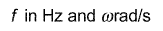The forward and inverse transforms for these two notational schemes are defined as: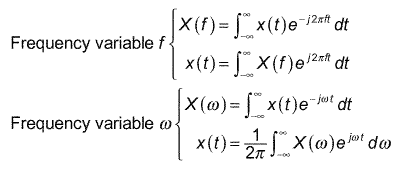. . . and here’s the table: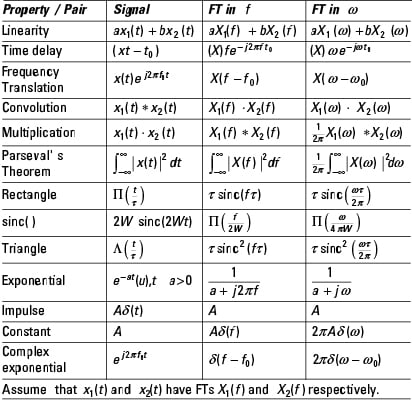## Applying Fourier transform to discrete-time signals

For discrete-time signals and systems the discrete-time Fourier transform (DTFT) takes you to the frequency domain. A short table of theorems and pairs for the DTFT can make your work in this domain much more fun. The discrete-time frequency variable is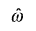The forward and inverse transforms are defined as: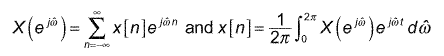. . . and here’s the table: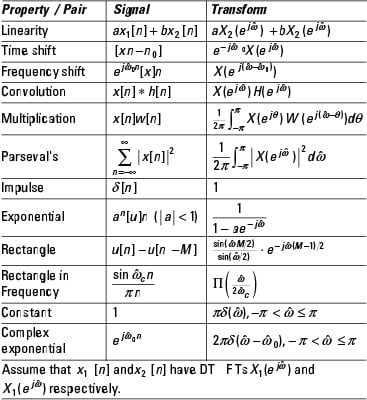## Using the Laplace transform in the s-domain

For continuous-time signals and systems, the one-sided Laplace transform (LT) helps to decipher signal and system behavior. It’s also the best approach for solving linear constant coefficient differential equations with nonzero initial conditions. The one-sided LT is defined as: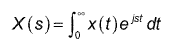The inverse LT is typically found using partial fraction expansion along with LT theorems and pairs. Here’s a short table of LT theorems and pairs.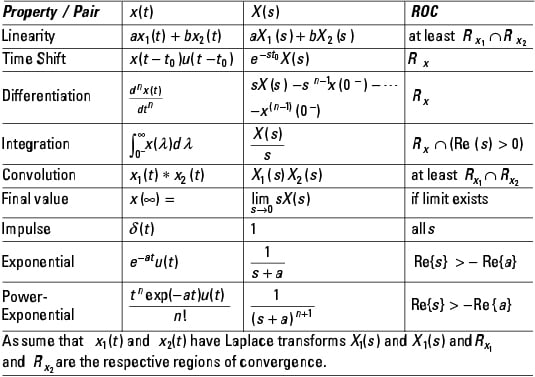## Letting the z-Transform help with signals and systems analysis

For discrete-time signals and systems, the z-transform (ZT) is the counterpart to the Laplace transform. With the ZT you can characterize signals and systems as well as solve linear constant coefficient difference equations. The two-sided ZT is defined as: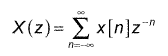The inverse ZT is typically found using partial fraction expansion and the use of ZT theorems and pairs. Here is a short table of ZT theorems and pairs.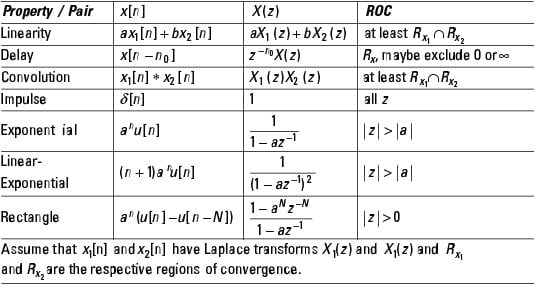## Exploring signals and systems: core concepts of sampling theory

Sampling theory links continuous and discrete-time signals and systems. For example, you can get a discrete-time signal from a continuous-time signal by taking samples every T seconds. This article points out some useful relationships associated with sampling theory. Key concepts include the low-pass sampling theorem, the frequency spectrum of a sampled continuous-time signal, reconstruction using an ideal lowpass filter, and the calculation of alias frequencies.

The table of properties begins with a block diagram of a discrete-time processing subsystem that produces continuous-time output y(t) from continuous-time input x(t). This block diagram motivates the sampling theory properties in the remainder of the table.

The process of converting continuous-time signal x(t) to discrete-time signal x[n] requires sampling, which is implemented by the analog-to-digital converter (ADC) block. The block with frequency response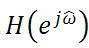represents a linear time invariant system with input x[n] and output y[n]. The discrete-time signal y[n] is returned to the continuous-time domain via a digital-to-analog converter and a reconstruction filter.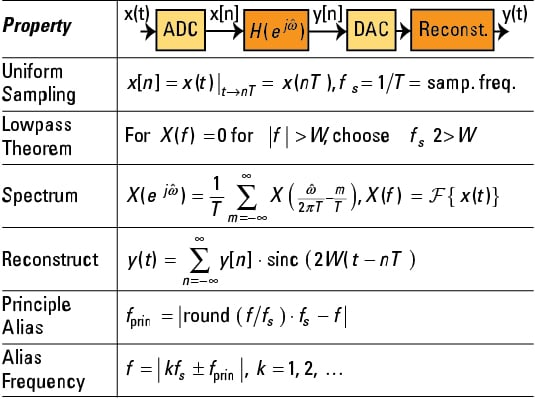## Synthesizing signals with the Fourier series

Periodic signals can be synthesized as a linear combination of harmonically related complex sinusoids. The theory of Fourier series provides the mathematical tools for this synthesis by starting with the analysis formula, which provides the Fourier coefficients Xn corresponding to periodic signal x(t) having period T0.

Common periodic signals include the square wave, pulse train, and triangle wave. This table shows the Fourier series analysis and synthesis formulas and coefficient formulas for Xn in terms of waveform parameters for the provided waveform sketches: